# Estimation: Introduction by Example¶

###### Click the button below to show/hide code¶
In :
from IPython.display import Latex
import matplotlib.pyplot as plt
%matplotlib inline
import numpy
import math
import scipy.misc
from numpy.linalg import pinv
from numpy.linalg import inv
from scipy.stats import norm
from scipy import integrate
from scipy.integrate import odeint
from IPython.display import Image


## In This Document¶

This document introduces some of the most common estimation techniques via example. We start with particle filters, and work our way to linear Kalman filters.

## Resources¶

Derivations for the EKF and ideas for explaining things come from Tucker McClure's website (http://www.anuncommonlab.com/articles/how-kalman-filters-work/). This is a fantastic resource, the only one of its kind that I've ever seen. You should read it and, if you like it, let Tucker know (he's a Cornell grad and a super nice guy).

Other resources for the other filters include lecture notes from Mark Psiaki's 6760 class, the book Applied Optimal Estimation by Gelb, and Optimal Control and Estimation by Stengel, and Estimation with Applications to Tracking and Navigatoin by Bar-Shalom.

## Introduction¶

Estimation is the practice of using noisy, limited, imperfect data to get the best possible estimate of some quantity of interest. Many of these techniques were born for aerospace and robotics applications and these are the most common places to find them in use, but they are general enough to be usable in a variety of other fields of study. All that we require is some model for the evolution of our system (an equation of motion), measurements of some quantity that is correlated with the quantity that we are trying to estimate, and an understanding of the noise present in our sensors and in our system. These are well understood in an aerospace/robotics context, but you can imagine modeling biological, oceanographic, geologic, or other systems and applying the same techniques.

Estimation is, in many ways, the mirror image of control. You can arrive at optimal estimation algorithms using precisely the same strategies used to arrive at optimal control methods. Your cost function, rather than minimizing the difference between a current and a desired state, instead minimizes the difference between the true and estimated state. We'll see this when we discuss the Kalman filter. Familiarity with control will make derivation of estimation algorithms easier, and vice versa.

In aerospace, the most common use for these estimation algorithms is to estimate the state of a spacecraft (quaternion, angular velocity, position, velocity) from sensors that are unable to detect these quantities directly, or that do so with a lot of noise. If you want to employ state feedback, then you're going to need to implement an estimation algorithm.

## NOTE!¶

The code in this document is written for clarity, not for efficiency. There are plenty of opportunities to optimize.

Also, every one of these filters could be made to perform better (in particular the nonlinear ones). These are just to provide some insight into how to construct these things.

## Dynamic System in Question¶

The point of this document is not dynamics, so I want to choose an example dynamical system that is as simple as possible while still illustrating the properties of the various estimators. Let us consider a ballistic projectile under the influence of drag and buffeting.

#### Equations of Motion¶

Consider the Lagrangian

\begin{align} \mathcal{L} &= T - V\\ &= \text{Kinetic Energy} - \text{Potential Energy} \end{align}

We'll use a set of cartesian coordinates with origin at the location of the cannon. In these coordinates, it is easy to write down the expressions for the kinetic and potential energy:

\begin{align} T &= \frac{1}{2}m\dot{x}^2 + \frac{1}{2}m\dot{y}^2 \end{align}
\begin{align} V &= mgy \end{align}

With these, we can write down the Lagrangian:

\begin{align} \mathcal{L} &= \frac{1}{2}m\dot{x}^2 + \frac{1}{2}m\dot{y}^2 - mgy \end{align}

Applying the Euler-Lagrange equation gives us two coupled differential equations that describe the motion of the spacecraft:

\begin{align} \frac{\partial{\mathcal{L}}}{\partial{q}} - \frac{d}{dt}\frac{\partial{\mathcal{L}}}{\partial{\dot{q}}} + Q_i = 0 \end{align}

In the above expression, $Q_i$ are the generalized forces acting on each generalized coordinate. In this case, we have only drag and buffeting (a random acceleration):

\begin{align} F_d &= -c\dot{x} \hat{x} - c\dot{y}\hat{y} \end{align}
\begin{align} F_b &= m\mathcal{N}(0,\sigma_{ax})\hat{x} + m\mathcal{N}(0,\sigma_{ay})\hat{y} \end{align}

Solving:

\begin{align} m\ddot{x} + c\dot{x} + m\mathcal{N}(0,\sigma_{ax}) &= 0 && m\ddot{y} + c\dot{y} - mg + m\mathcal{N}(0,\sigma_{ay}) = 0 \end{align}

#### State-Space Model¶

Get these differential equations into a state-space representation:

\begin{align} q_1 &= x && \dot{q_1} = \dot{x} = q_2\\ q_2 &= \dot{x} && \dot{q_2} = \ddot{x} = -\frac{c}{m}\dot{x} + \mathcal{N}(0,\sigma_{ax})\\ q_3 &= y && \dot{q_3} = \dot{y} = q_4\\ q_4 &= \dot{y} && \dot{q_4} = \ddot{y} = -\frac{c}{m}\dot{y} + g + \mathcal{N}(0,\sigma_{ay}) \end{align}

#### Simulation¶

Create some constants and initial conditions

\begin{align} g &= \text{gravitational acceleration} = 9.81m/s \\ m &= \text{projectile mass} = 1 kg\\ c &= \text{damping constant} = 2 kg/s\\ T &= \text{length of simulation} = 15sec\\ n &= \text{number of simulation steps} = 100\\ t &= \text{array of times}\\ \Delta t &= \text{size of timestep} = \frac{T}{n}\\ \sigma_x &= \text{standard deviation of gaussian buffeting in $x$} = 1 m/s^2\\ \sigma_y &= \text{standard deviation of gaussian buffeting in $y$} = 1 m/s^2\\ x_0 &= \begin{bmatrix} 50 & 80 & 10 & 0\end{bmatrix} \end{align}
In :
g=9.81
m=1
c=2.
x0 = [50, 80, 10, 0]
stop = 15
numsteps = 100.
t = numpy.linspace(0,stop,numsteps)
delta_t = stop/numsteps
sigx = 1
sigy = 1
x_rand = sigx*numpy.random.randn(len(t))
y_rand = sigy*numpy.random.randn(len(t))


Functions for integrating the system. These functions will integrate the system of differential equations shown below, derived above, using some standard Python tools.

\begin{align} q_1 &= x && \dot{q_1} = \dot{x} = q_2\\ q_2 &= \dot{x} && \dot{q_2} = \ddot{x} = -\frac{c}{m}\dot{x} + \mathcal{N}(0,\sigma_{ax})\\ q_3 &= y && \dot{q_3} = \dot{y} = q_4\\ q_4 &= \dot{y} && \dot{q_4} = \ddot{y} = -\frac{c}{m}\dot{y} + g + \mathcal{N}(0,\sigma_{ay}) \end{align}
In :
def derivatives(X, t):
x, y, xdot, ydot = X
noise_x = x_rand[int(t/delta_t)-2]
noise_y = y_rand[int(t/delta_t)-2]
return [xdot+delta_t*noise_x, ydot+delta_t*noise_y,
-(c/m)*xdot+noise_x, -(c/m)*ydot - g + noise_y]

def noiselessDerivatives(X, t):
x, y, xdot, ydot = X
return [xdot, ydot,
-(c/m)*xdot, -(c/m)*ydot - g]

def integrate():
sol = odeint(derivatives, x0, t)
return sol


We will also find it useful to have a function like those that integrate the full simulation, but that stops after one timestep. We can pass this function the current state and it will integrate one timestep and return the state at the next timestep (assuming zero noise).

In :
def f(state):
return odeint(noiselessDerivatives, state, numpy.arange(0, 2*delta_t, delta_t))[-1]


With these constants, functions, and initial conditions defined, let's integrate the equations of motion to create a trajectory. I will store that time history in an array so that it can be used for filter testing.

In :
trajectory = integrate()
x_t, y_t, xd_t, yd_t = trajectory[:,0], trajectory[:,1] ,trajectory[:,2], trajectory[:,3]
true_trajectory = numpy.vstack((x_t,y_t,xd_t,yd_t))

In :
def showTrajectory(results):
plt.plot(trajectory[:,0], trajectory[:,1], label='trajectory')
plt.title('Projectile Trajectory')
plt.xlabel('meters'); plt.ylabel('meters')
plt.legend(loc='best')
plt.ylim([0,100])
plt.show()
plt.plot(t, trajectory[:,0])
plt.title('Projectile x-Position')
plt.xlabel('sec'); plt.ylabel('meters')
plt.show()
plt.plot(t, trajectory[:,1])
plt.title('Projectile y-Position')
plt.xlabel('sec'); plt.ylabel('meters')
plt.show()
plt.plot(t, trajectory[:,2])
plt.title('Projectile x-Velocity')
plt.xlabel('sec'); plt.ylabel('meters/sec')
plt.show()
plt.plot(t, trajectory[:,3])
plt.title('Projectile y-Velocity')
plt.xlabel('sec'); plt.ylabel('meters/sec')
plt.show()
showTrajectory(trajectory)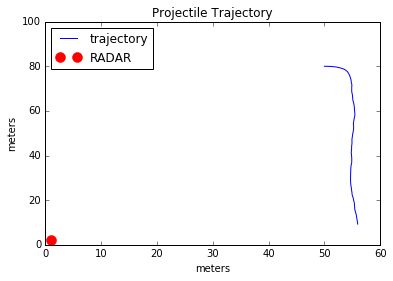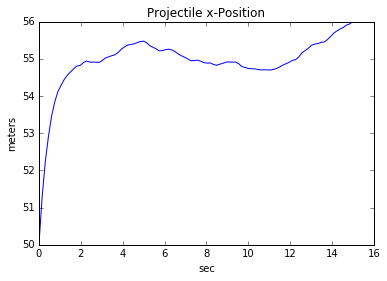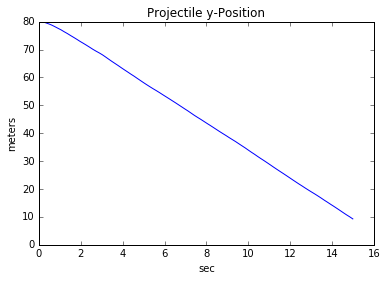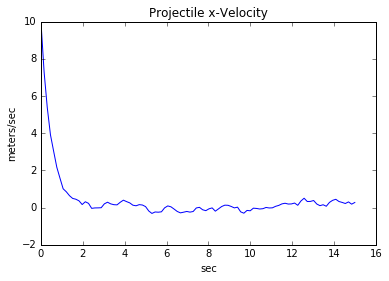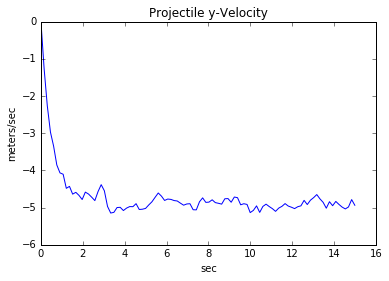The projectile starts with some velocity in the positive x-direction, which is quickly damped out due to drag. The projectile than falls down, getting unpredictably buffetted in all directions as it falls. This is the type of path that you might expect for a tennis ball falling through stormy weather.

## Measurement Model¶

We want to estimate the time-varying position and velocity of the projectile. In order to do that, all that we need is a series of measurements of a quantity (or different quantities) that are correlated with the position and velocity. Obviously, if we had a GPS then this would give us the information that we needed. Let's make it slightly more complicated though, just to illustrate some features of the estimators.

Let us assume that we have a RADAR sitting on the origin that measures distance to the projectile, as shown in the plot above. It does not tell us the angular location of the projectile, or its velocity. It just tells us how far away the object is at a given moment in time.

If our measurement is $z$ and our state is ${\bf{x}} = \begin{bmatrix} x & y & \dot{x} & \dot{y}\end{bmatrix}$, then our measurements relate to our state according to the following equation:

\begin{align} z = h({\bf{x}}) = \sqrt{x^2 + y^2} + w \end{align}
In :
def h(state):
x, y, xdot, ydot = state
return numpy.sqrt(x**2. + y**2.)


where $w$ is the measurment noise, a zero-mean Gaussian random number with known variance R ($w \sim \mathcal{N}(0,R)$). We can simulate these measurements for the trajectory shown above.

Define $R$. In practice, this is a number that would come from the datasheet of your sensor. Let's assume a value of $1$. That is to say, the noise on the radar measurements is zero-mean and Gaussian, with a standard deviation of 1.

In :
R = 1.


Using the measurement equation above, and the time history of the true trajectory of the projectile, we can generate a series of radar measurements associated with that trajectory. These are generated by passing the state at each timestep through the measurement equation and adding some Gaussian noise with the appropriate standard deviation. The measurements for the entire trajectory are shown below.

In :
measurements = h(true_trajectory[:,:]) + R*numpy.random.randn(len(t))

In :
def showMeasurements(meas):
plt.plot(t, measurements, label='Ranging measurements')
plt.title("Ranging Measurements")
plt.xlabel('sec'); plt.ylabel('meters')
plt.legend(loc='best')
plt.show()
showMeasurements(measurements)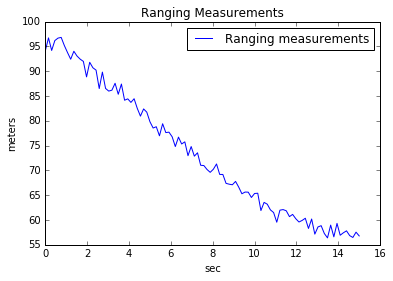## Quick Pause¶

Let's review what we have. We have an array of the true trajectory of the projectile, which we are trying to estimate (obviously, in the real world you never know the true values for the quantities being estimated, we can generate them in simulation to study filter performance).

In :
true_trajectory;


We have an array of measurements, which we will use to estimate the trajectory. We could produce these sequentially as the filter runs, but it's good practice to batch them. This reduces the number of computations required for filter testing, speeding up the process.

In :
measurements;


We have a function ${\bf{f}}({\bf{x}})$ that takes a state as an input, and returns the state at the next timestep:

In :
f(x0)

Out:
array([ 51.29590889,  79.89989331,   7.40818221,  -1.27128663])

We have a function ${\bf{h}}({\bf{x}})$ which takes a state as an input, and returns the measurement that one would expect for that state:

In :
h(x0)

Out:
94.339811320566042

We have the error covariance for our measurments, $R$.

In :
R

Out:
1.0

And we have the error covariance for our process, $Q$ (in the real world, this can be a very difficult quantity to know).

In :
Q = numpy.array([[.25*(delta_t**4.)*sigx, 0., .5*(delta_t**3.)*sigx, 0.],
[0., .25*(delta_t**4.)*sigy, 0., .5*(delta_t**3.)*sigy],
[.5*(delta_t**3.)*sigx, 0., (delta_t**2.)*sigx, 0.],
[0., .5*(delta_t**3.)*sigy, 0., (delta_t**2.)*sigy]])
Q=Q+numpy.eye(4)*(1e-12)


This is everything that we need for the first two types of filters: the particle filter and the UKF.

## The Particle Filter¶

### Intuition¶

At the highest level, the particle filter operates by asking the following questions:

1. What are all the things that could possibly be happening?
2. If each of those things were happening, what measurements would I expect?
3. Given an actual measurement, how likely is it that each of these things are happening?

The particle filter uses computation to overpower uncertainty in the system. In the case of the projectile, we have uncertainty about the initial position and velocity and uncertainty in the dynamics due to buffeting. The particle filter's solution is to simply simulate hundreds or thousands of projectiles at each timestep, figure out the measurements that you would get for each of those simulated projectiles, and compare those measurements to the real measurements. The estimate is simply a weighted average of all of the particles (weighted by how well their simulated measurements match the real measurements), and the covariance is calculated numerically by measuring how spread-out the particles are.

### Outline of Algorithm¶

#### A. Start with an initial estimate for the state, ${\bf{\hat{x}}(0)}$, and an initial estimate for the covariance, $P(0)$.¶

\begin{align} {\bf{\hat{x}(0)}} &= \text{Initial state estimate}\\ P(0) &= \text{Initial covariance estimate (how confident are you in your initial guess?)} \end{align}

It's worth pausing briefly to reinforce the idea of covariance. This is one of those simple concepts that it often made to seem less intuitive than it really is. Let us consider a random variable $a$. The Expected Value for $a$ is simply the mean of many, many samples of $a$. So, the expected value for a roll of a die is 3.5. Here's another way to think about it. Suppose that $a$ is a random variable drawn from the probability density function shown below:

In :
def drawPDF(e=.8):
theta = numpy.linspace(0, 2*numpy.pi, 100)
p = ((1-e)**(3./2))/(2*numpy.pi*(1/(e+1))**(3./2)*(e*numpy.cos(theta)+1)**2.)
plt.plot(theta, p);
plt.xlabel('a');plt.ylabel('$p(x)$');
plt.title('Probability Density Function for $a$');plt.show()
drawPDF()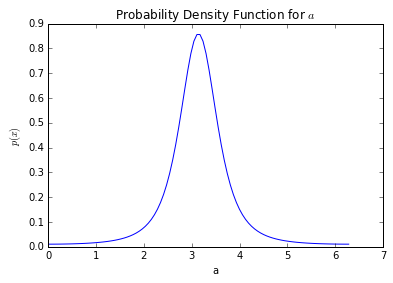Visually, the Expected Value for $a$ is the x-coordinate of the center of mass (the balance point) of this disribution. In this case, you can see that the expected value for $a$ is at 3.14. In fact, this is the probability density function for the angular position of a spacecraft in elliptical orbit, measured from periapsis. So this is saying that the expected value for the position of a spacecraft is apoapsis, which makes sense. Let's define $\overline{a}$ to be the expected value for the random variable $a$.

The Variance of the variable $a$ is the expected value of a different random variable. That random variable is $\left(a - \overline{a}\right)^2$, the square deviation of the variable $a$ from the mean of the distribution.

\begin{align} \sigma_a^2 &= E\left[(a - \overline{a})^2\right] \end{align}

Covariance is just the multivariate extension of this concept. Suppose we have a vector of random variables $c = \begin{bmatrix} a & b\end{bmatrix}$. Then the covariance is given by:

\begin{align} P_{cc} &= \begin{bmatrix} E\left[(a - \overline{a})^2\right] & E\left[(a - \overline{a})(b - \overline{b})\right]\\ E\left[(b - \overline{b})(a - \overline{a})\right] & E\left[(b - \overline{b})^2\right] \end{bmatrix} \end{align}

On the diagonals, we simply have the variance of each random variable in the vector. On the off-diagonals, we have the covariance of each of these variables (is it obvious that this is a symmetric matrix?). If the random variables $a$ and $b$ were independent rolls of two die, the off diagonals would be zero. This is because the two variables are uncorrelated. These are nonzero if there is correlation between the two random variables.

#### B. Decide how many particles will be in your particle filter (100? 1000? 10000?). Call this number $N_s$.¶

\begin{align} N_s &= \text{Number of particles} \end{align}

#### D. Create $N_s$ particles by sampling $N_s$ times from the distribution $\mathcal{N}({\bf{\hat{x}(0)}},P(0))$¶

\begin{align} \underline{\chi}^i(0) &\sim \mathcal{N}({\bf{\hat{x}(0)}},P(0)) \end{align}

#### E. Create an array of length $N_s$ of weights. Initially, each weight is $\frac{1}{N_s}$, since no particle is more likely than any other.¶

\begin{align} \underline{w}_s &= \frac{1}{N_s} \end{align}

#### F. Sample an array of random variables $\underline{v}^i(k)$ from $\mathcal{N}(0,Q)$ to generate $i=1\dots N_s$ process noises.¶

\begin{align} \underline{v}^i(k) &\sim \mathcal{N}(0,Q) \end{align}

#### G. Integrating the nonlinear dynamic equations, propagate each particle forward one timestep.¶

\begin{align} \underline{\chi}^i(k+1) &= {\bf{f}}(k, \underline{\chi}^i(k), \underline{u}(k), \underline{v}^i(k)) \end{align}

#### H. Using the measurement equations, find the measurements that you would expect for each of these particles.¶

\begin{align} \underline{\xi}^i &= {\bf{h}}(k+1,\underline{\chi}^i(k+1)) \end{align}

#### I. Gather an actual measurement, $z(k+1)$. Find the difference between the real measurement and each particle's simulated measurement.¶

\begin{align} \underline{\nu}^i &= \underline{\xi}^i - z(k+1) \end{align}

#### J. Weight each particle according to how well it matches the true measurements. NOTE: There are many ways to do this, and different ways work better for different problems. Here is one method that tends to work well.¶

We square the differences between measurements, scaling by the measurement error covariance matrix, and add the log of the previous weight. These weights are then normalized to the largest weight. This prevents one particle from stealing all the weight too quickly.

\begin{align} log(\underline{w}(k+1)) &= -\frac{1}{2}\left(\underline{\nu}^i\right)^TR^{-1}\left(\underline{\nu}^i\right) + log(\underline{w}(k))\\ log(\underline{w}(k+1))^{MAX} &= max(log(\underline{w}(k+1)))\\ \tilde{\underline{w}}(k+1) &= exp(log(\underline{w}(k+1)) - log(\underline{w}(k+1))^{MAX})\\ \underline{w}(k+1) &= \frac{\tilde{\underline{w}}(k+1)}{\sum_{l=1}^{N_s}\tilde{w}_l(k+1)} \end{align}

#### K. Update state estimate (just a weighted average)¶

\begin{align} {\bf{\hat{x}}}(k+1) &= \sum_{i=1}^{N_s} w_i(k+1) \xi_i(k+1) \end{align}

#### L. Update covariance estimate (weighted version of standard numeric variance calculation)¶

Based on the covariance discussion above, does this make sense as a numerical approximation to the covariance?

\begin{align} P(k+1) &= \sum_{i=1}^{N_s}w_i(k+1)\left[\chi_i(k+1) - {\bf{\hat{x}}}(k+1)\right]\left[\chi_i(k+1) - {\bf{\hat{x}}}(k+1)\right]^T \end{align}

#### M. Compute the effective number of particles:¶

\begin{align} N_{eff} &= \frac{1}{\sum_{i=1}^{N_s}(w_i(k+1)^2)} \end{align}

### One Iteration¶

This walks through the first iteration of the particle filter (from the zeroth to the first timestep) and plots the relative quantities so that you can see what is happening.

#### Set the number of particles, and the threshold number of particles¶

\begin{align} N_s &= 100\\ N_t &= 8 \end{align}
In :
Ns=100
Nt=8


#### Initial covariance and state estimate¶

\begin{align} P(0) &= \begin{bmatrix} 0.1 & 0 & 0 & 0\\ 0 & 0.1 & 0 & 0\\ 0 & 0 & 0.01 & 0\\ 0 & 0 & 0 & 0.01 \end{bmatrix} x(0) &= x_0 + \mathcal{N}(0,P(0)) \end{align}

where $x_0$ is from the dynamics simulation above.

In :
P0 = numpy.array([[.1, 0., 0., 0.],
[0., .1, 0., 0.],
[0., 0., .01, 0.],
[0., 0., 0., .01]])
x_start = x0 + numpy.random.multivariate_normal(numpy.zeros(4), P0)


#### Initialize Weights¶

\begin{align} \underline{w}_s &= \frac{1}{N_s} \end{align}
In :
def resetWeights():
ws = numpy.ones(Ns)*(1./Ns)
logweights = numpy.log(ws)
return ws, logweights
ws, logweights = resetWeights()


#### Create Particles¶

\begin{align} \underline{\chi}^i(0) &\sim \mathcal{N}({\bf{\hat{x}(0)}},P(0)) \end{align}
In :
def resetParticles(x, P):
particles_k = numpy.random.multivariate_normal(x, P, Ns)
return particles_k
first_particles = resetParticles(x_start, P0)
plt.plot(first_particles[:,0], first_particles[:,1], 'b.', alpha=0.2, label='particles')
plt.plot(true_trajectory[0,0], true_trajectory[1,0], 'r*', label='true position')
plt.plot(x_start, x_start, 'g*', label='initial estimate')
plt.legend(loc='best')
plt.show()

---------------------------------------------------------------------------
NameError                                 Traceback (most recent call last)
<ipython-input-4-7045548d2726> in <module>()
2     particles_k = numpy.random.multivariate_normal(x, P, Ns)
3     return particles_k
----> 4 first_particles = resetParticles(x_start, P0)
5 plt.plot(first_particles[:,0], first_particles[:,1], 'b.', alpha=0.2, label='particles')
6 plt.plot(true_trajectory[0,0], true_trajectory[1,0], 'r*', label='true position')

NameError: name 'x_start' is not defined

#### Create Process Noises¶

\begin{align} \underline{v}^i(k) &\sim \mathcal{N}(0,Q) \end{align}
In :
def resetNoise():
noise_k = numpy.random.multivariate_normal(numpy.zeros(4), Q, Ns)
return noise_k
noise = resetNoise()


#### Propagate all Particles¶

\begin{align} \underline{\chi}^i(k+1) &= {\bf{f}}(k, \underline{\chi}^i(k), \underline{u}(k), \underline{v}^i(k)) \end{align}
In :
def propagateParticles(particles, noise):
new_particles = numpy.zeros((Ns, 4))
for i in range(Ns):
new_particles[i,:] = f(particles[i,:] + noise[i,:])
return new_particles
new_particles = propagateParticles(first_particles, noise)
plt.plot(new_particles[:,0], new_particles[:,1], 'b.', alpha=0.5, label='new particles')
plt.plot(first_particles[:,0], first_particles[:,1], 'b.', alpha=0.2, label='particles')
plt.plot(true_trajectory[0,0], true_trajectory[1,0], 'r*', alpha=0.5)
plt.plot(true_trajectory[0,1], true_trajectory[1,1], 'r*', label='true position')
plt.plot(x_start, x_start, 'g*', label='initial estimate')
plt.legend(loc='best')
plt.show()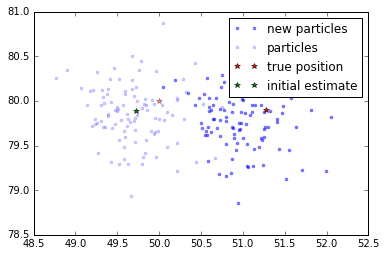#### Update Weights¶

\begin{align} log(\underline{w}(k+1)) &= -\frac{1}{2}\left(\underline{\nu}^i\right)^TR^{-1}\left(\underline{\nu}^i\right) + log(\underline{w}(k))\\ log(\underline{w}(k+1))^{MAX} &= max(log(\underline{w}(k+1)))\\ \tilde{\underline{w}}(k+1) &= exp(log(\underline{w}(k+1)) - log(\underline{w}(k+1))^{MAX})\\ \underline{w}(k+1) &= \frac{\tilde{\underline{w}}(k+1)}{\sum_{l=1}^{N_s}\tilde{w}_l(k+1)} \end{align}
In :
def updateWeights(index, new_particles, logweights):
measurement = measurements[index]
for i in range(Ns):
logweights[i] = (-.5*(measurement - h(new_particles[i,:]))*(1./R)*
(measurement - h(new_particles[i,:])) + logweights[i])
maxlog = max(logweights)
intermediate_weights = numpy.exp(logweights - maxlog)
new_weights = intermediate_weights/(numpy.sum(intermediate_weights))
return logweights, new_weights

logweights, ws = updateWeights(1, new_particles, logweights)
plt.plot(true_trajectory[0,0], true_trajectory[1,0], 'r*', alpha=0.5)
plt.plot(true_trajectory[0,1], true_trajectory[1,1], 'r*', label='true position')
plt.plot(x_start, x_start, 'g*', label='initial estimate')
alphas = ws/max(ws)
for i in range(Ns):
plt.plot(new_particles[i,0], new_particles[i,1], 'b.', alpha=alphas[i])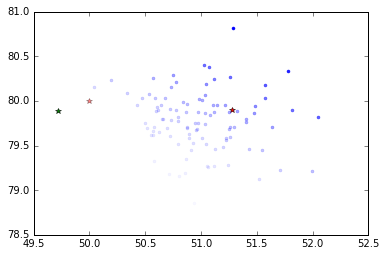#### Compute Estimate¶

\begin{align} {\bf{\hat{x}}}(k+1) &= \sum_{i=1}^{N_s} w_i(k+1) \xi_i(k+1) \end{align}
\begin{align} P(k+1) &= \sum_{i=1}^{N_s}w_i(k+1)\left[\xi_i(k+1) - {\bf{\hat{x}}}(k+1)\right]\left[\xi_i(k+1) - {\bf{\hat{x}}}(k+1)\right]^T \end{align}
In :
def computeEstimate(weights, particles):
x_new = numpy.zeros(4)
P_new = numpy.zeros((4,4))
for i in range(Ns):
x_new += weights[i]*particles[i,:]
for i in range(Ns):
diff = numpy.array([(particles[i,:] - x_new)])
P_new += weights[i]*numpy.dot(diff.T, diff)
return x_new, P_new

new_est, new_P = computeEstimate(ws, new_particles)
plt.plot(true_trajectory[0,0], true_trajectory[1,0], 'r*', alpha=0.5)
plt.plot(true_trajectory[0,1], true_trajectory[1,1], 'r*', label='true position')
plt.plot(x_start, x_start, 'g*', alpha=0.5, label='initial estimate')
plt.plot(new_est, new_est, 'g*', label='updated estimate')
alphas = ws/max(ws)
for i in range(Ns):
plt.plot(new_particles[i,0], new_particles[i,1], 'b.', alpha=alphas[i])
print new_P

[[ 0.16128263 -0.00261716  0.00662438 -0.0043407 ]
[-0.00261716  0.1134494   0.00113107  0.00625394]
[ 0.00662438  0.00113107  0.01899366 -0.00029339]
[-0.0043407   0.00625394 -0.00029339  0.01451163]]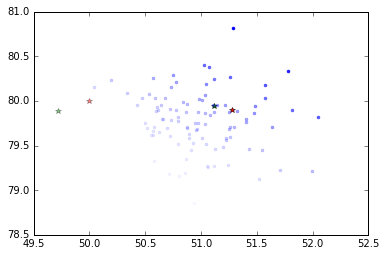#### Compute the effective number of particles¶

\begin{align} N_{eff} &= \frac{1}{\sum_{i=1}^{N_s}(w_i(k+1)^2)} \end{align}
In :
def effectiveParticles(weights):
return 1/(numpy.sum(weights*weights))

print effectiveParticles(ws)

68.0062350571


### Full Simulation¶

Run the particle filter over the whole trajectory.

In :
def runParticleFilter():
P = P0
x_start = x0 + numpy.random.multivariate_normal(numpy.zeros(4), P0)
ws, logweights = resetWeights()
particles_k = resetParticles(x_start, P)
noise = resetNoise()
Neff = Ns

P_array = numpy.zeros((len(t), 4, 4))
x_array = numpy.zeros((len(t), 4))
P_array = P
x_array[0,:] = x_start
for i in range(1, len(t)):
new_particles = propagateParticles(particles_k, noise)
logweights, ws = updateWeights(i, new_particles, logweights)
x_new, P_new = computeEstimate(ws, new_particles)
Neff = effectiveParticles(ws)

P_array[i] = P_new
x_array[i,:] = x_new

if Neff < 5:
particles_k = resetParticles(x_new, P_new)
noise = resetNoise()
ws, logweights = resetWeights()
else:
particles_k = new_particles
noise = resetNoise()
return x_array, P_array
pfresults = runParticleFilter()
pf_traj, pf_cov = pfresults


### Show Results¶

Plot the results.

In :
def showPFResults(trajectory, pf_traj, pf_cov):
plt.plot(trajectory[:,0], trajectory[:,1], label='true trajectory')
plt.plot(pf_traj[:,0], pf_traj[:,1], label='estimated trajectory')
plt.title('True and Estimated Projectile Trajectory')
plt.xlabel('meters'); plt.ylabel('meters')
plt.legend(loc='best')
plt.ylim([0,100])
plt.show()
plt.plot(t, trajectory[:,0], label='true')
plt.plot(t, pf_traj[:,0], label='estimated')
plt.legend(loc='best')
plt.title('Projectile x-Position')
plt.xlabel('sec'); plt.ylabel('meters')
plt.show()
plt.plot(t, trajectory[:,1], label='true')
plt.plot(t, pf_traj[:,1], label='estimated')
plt.legend(loc='best')
plt.title('Projectile y-Position')
plt.xlabel('sec'); plt.ylabel('meters')
plt.show()
plt.plot(t, trajectory[:,2], label='true')
plt.plot(t, pf_traj[:,2], label='estimated')
plt.legend(loc='best')
plt.title('Projectile x-Velocity')
plt.xlabel('sec'); plt.ylabel('meters/sec')
plt.show()
plt.plot(t, trajectory[:,3], label='true')
plt.plot(t, pf_traj[:,3], label='estimated')
plt.legend(loc='best')
plt.title('Projectile y-Velocity')
plt.xlabel('sec'); plt.ylabel('meters/sec')
plt.show()

x_err = trajectory[:,0] - pf_traj[:,0]
y_err = trajectory[:,1] - pf_traj[:,1]
xdot_err = trajectory[:,2] - pf_traj[:,2]
ydot_err = trajectory[:,3] - pf_traj[:,3]

xsig, ysig, xdotsig, ydotsig = [], [], [], []
for i in range(len(pf_cov)):
xsig.extend([numpy.sqrt(pf_cov[i])])
ysig.extend([numpy.sqrt(pf_cov[i])])
xdotsig.extend([numpy.sqrt(pf_cov[i])])
ydotsig.extend([numpy.sqrt(pf_cov[i])])
plt.plot(x_err, 'b-', label='error')
plt.plot(3*numpy.array(xsig), 'r-', label='3 sigma')
plt.plot(-3*numpy.array(xsig), 'r-', label='3 sigma')
plt.legend(loc='best')
plt.show()
plt.plot(y_err, 'b-', label='error')
plt.plot(3*numpy.array(ysig), 'r-', label='3 sigma')
plt.plot(-3*numpy.array(ysig), 'r-', label='3 sigma')
plt.legend(loc='best')
plt.show()
plt.plot(xdot_err, 'b-', label='error')
plt.plot(3*numpy.array(xdotsig), 'r-', label='3 sigma')
plt.plot(-3*numpy.array(xdotsig), 'r-', label='3 sigma')
plt.legend(loc='best')
plt.show()
plt.plot(ydot_err, 'b-', label='error')
plt.plot(3*numpy.array(ydotsig), 'r-', label='3 sigma')
plt.plot(-3*numpy.array(ydotsig), 'r-', label='3 sigma')
plt.legend(loc='best')
plt.show()
showPFResults(trajectory, pf_traj, pf_cov)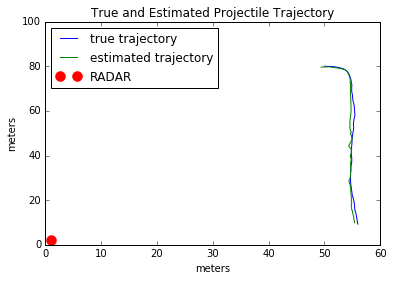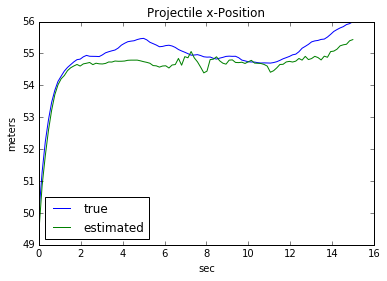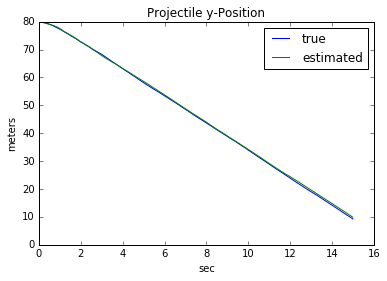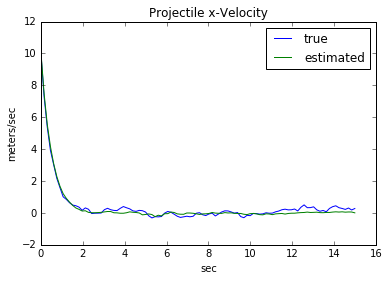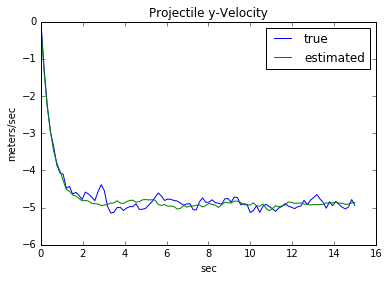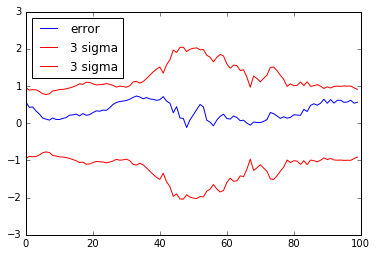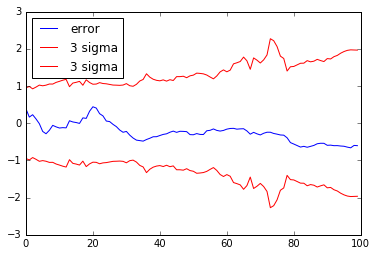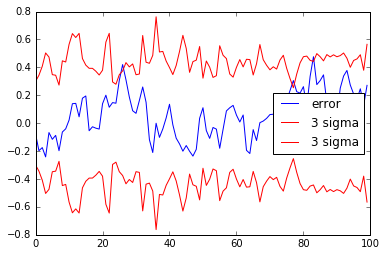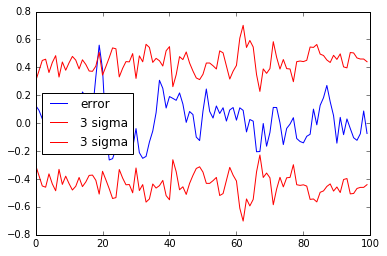### Finishing Thoughts¶

Pros: Easy to implement (doesn't require any Jacobians), tunable to specific problems by modifying resampling methods, can be use on multimodal probability density functions, very little theoretical work required. Also, this supports any kind of distribution, not just Gaussian.

Cons: Requires lots of particles (computationally expensive), often difficult to get the same degree of accuracy as other estimation methods, might take a lot of tweaking to get it behaving properly for your application.

## The Sigma-Point (Unscented Kalman) Filter¶

### Intuition: Error Covariance and Inertia Tensors¶

What if we knew (or assumed) that the uncertainty in our estimate were Gaussian? In that case, we could represent the uncertainty as a covariance matrix. Rather than splashing tons of particles at the problem, we can take advantage of the Gaussian nature of our uncertainty (and the Gaussian nature of the process noise) in order to pick just a few particles very strategically.

This is precisely what the UKF, or Sigma Point Filter, does. In almost every way, it is exactly like a particle filter. The estimates are formed by doing simple weighted averages, the covariances are calculated numerically, and each particle (now called a "sigma point") is propagated through the full nonlinear dynamic equations. The only difference is that our particles are no longer random draws, but strategic draws based upon the underying distributions for our uncertainty and process noise. Where should these particles go? One stays exactly on the current estimate. But what about the others?

Covariance matrices are symmetric and positive (semi) definite. Does that sound like any other matrices that you are familiar with? Answer: inertia tensors.

The others are placed along the principle axes of the covariance matrix, at a distance that is tunable by the filter designer. If you were interpreting your error covariance matrix as an inertia tensor, your sigma points would be along the principle axes of the rigid body. The eigenvectors of the covariance matrix point along the principle axes, the square roots of the eigenvalues are the semi-axis lengths of the corresponding ellipsoid.

### Outline of Algorithm¶

#### A. Start at sample k with ${\bf{\hat{x}}}(k)$, $P(k)$, $Q(k)$ known. Set tuning parameters $\alpha$ and $\beta$.¶

\begin{align} \text{State Estimate} &= {\bf{\hat{x}}}(k)\\ \text{Coveriance Estimate} &= P(k)\\ \text{Process Noise} &\sim \mathcal{N}(0,Q(k))\\ \alpha &= \text{Tuning parameter}, 10^{-4}<\alpha<1\\ \beta &= \text{Tuning parameter}, \beta=2 \text{ optimal for Gaussian} \end{align}

#### B. Cholesky factorize P(k) and Q(k) (essentially a matrix square root).¶

\begin{align} P(k) &= S_x(k)S_x(k)^T\\ &\rightarrow S_x(k) = \text{chol}(P(k))\\ Q(k) &= S_v(k)S_v(k)^T\\ &\rightarrow S_v(k) = \text{chol}(Q(k)) \end{align}

#### C. Define individual columns of these covariance square roots:¶

\begin{align} S_x(k) &= \begin{bmatrix} \underline{s}_{x_1k} & \underline{s}_{x_2k} & \cdots & \underline{s}_{n_xk}\end{bmatrix}\\ S_xvk) &= \begin{bmatrix} \underline{s}_{v_1k} & \underline{s}_{v_2k} & \cdots & \underline{s}_{n_vk}\end{bmatrix} \end{align}

#### D. Generate the following $1 + 2(n_x + n_v)$ sigma points:¶

\begin{align} \chi_k^0 &= {\bf{\hat{x}}}(k) && v_k^0 = 0 && i=0\\ \chi_k^i &= {\bf{\hat{x}}}(k) + \underline{s}_{x_ik} \sqrt{n_x + n_v + \lambda} && v_k^i=0 && i=1,\dots,n_x\\ \chi_k^i &= {\bf{\hat{x}}}(k) - \underline{s}_{x_{(i-n_x)}k} \sqrt{n_x + n_v + \lambda} && v_k^i=0 && i=n_x+1,\dots,2n_x\\ \chi_k^i &= {\bf{\hat{x}}}(k) && v_k^i=\underline{s}_{v_{(i-2n_x)}k} \sqrt{n_x + n_v + \lambda} && i=2n_x + 1,\dots,2n_x + n_v\\ \chi_k^i &= {\bf{\hat{x}}}(k) && v_k^i=-\underline{s}_{v_{(i-2n_x - n_v)}k} \sqrt{n_x + n_v + \lambda} && i=2n_x+n_v+1,\dots,2(n_x+n_v)\\ \end{align}

where $\lambda$ is a scaling parameter that is tunable.

\begin{align} \lambda &= \alpha^2(n_x + \kappa) - n_x\\ \kappa &= 3-n_x \end{align}

#### E. Integrating the nonlinear dynamic equations, propagate each sigma point forward one timestep.¶

\begin{align} \underline{\chi}^i(k+1) &= {\bf{f}}(k, \underline{\chi}^i(k), \underline{u}(k), \underline{v}^i(k)) \end{align}

#### F. Using the measurement equations, find the measurements that you would expect for each of these particles.¶

\begin{align} \underline{\xi}^i &= {\bf{h}}(k+1,\underline{\chi}^i(k+1)) \end{align}

#### G. Calculate the weights for the state estimate. Weight for the zeroth sigma point:¶

\begin{align} w_0^m &= \frac{\lambda}{n_x + n_v + \lambda} \end{align}

And the weights for all other sigma points:

\begin{align} w_{i\neq 0}^m &= \frac{1}{2(n_x + n_v + \lambda)} \end{align}

#### H. Use these weights to calculate the expected estimate (not measurement corrected) and the expected mean measurement:¶

\begin{align} \overline{x}(k+1) &= \sum_{i=0}^{2(n_x + n_v)}w_i^m \chi^i(k+1)\\ \overline{z}(k+1) &= \sum_{i=0}^{2(n_x + n_v)}w_i^m \xi^i(k+1) \end{align}

#### I. Calculate the weights for the covariance estimate. Weight for zeroth sigma point:¶

\begin{align} w_0^c &= \frac{\lambda}{n_x + n_v + \lambda} + 1 - \alpha^2 + \beta \end{align}

And all other sigma point weights:

\begin{align} w_{i\neq 0}^c &= \frac{1}{2(n_x + n_v + \lambda)} \end{align}

#### J. Calculate covariances (expected residual covariance, state covariance, and cross covariance)¶

\begin{align} \overline{P}(k+1) &= \sum_{i=0}^{2(n_x+n_v)} w_i^c \left[\chi^i(k+1) - \overline{x}(k+1)\right]\left[\chi^i(k+1) - \overline{x}(k+1)\right]^T\\ \overline{P}_{xz}(k+1) &= \sum_{i=0}^{2(n_x+n_v)} w_i^c \left[\chi^i(k+1) - \overline{x}(k+1)\right]\left[\xi^i(k+1) - \overline{z}(k+1)\right]^T\\ \overline{P}_{zz}(k+1) &= \sum_{i=0}^{2(n_x+n_v)} w_i^c \left[\xi^i(k+1) - \overline{z}(k+1)\right]\left[\xi^i(k+1) - \overline{z}(k+1)\right]^T \end{align}

#### K. Compute the Kalman Gain¶

\begin{align} K &= \overline{P}_{xz}\overline{P}_{zz}^{-1} \end{align}

#### L. Take a measurement, and use the linear minimum mean square error estimate form to compute the new state and covariance estimates¶

\begin{align} {\bf{\hat{x}}}(k+1) &= \overline{x}(k+1) + K\left[z(k+1) - \overline{z}(k+1)\right]\\ P(k+1) &= \overline{P}(k+1) - KRK^T \end{align}

### One Iteration¶

This walks through the first iteration of the UKF (from the zeroth to the first timestep) and plots the relative quantities so that you can see what is happening.

#### Initial covariance and state estimate¶

\begin{align} \text{State Estimate} &= {\bf{\hat{x}}}(k)\\ \text{Coveriance Estimate} &= P(k)\\ \text{Process Noise} &\sim \mathcal{N}(0,Q(k))\\ \alpha &= \text{Tuning parameter}, 10^{-4}<\alpha<1\\ \beta &= \text{Tuning parameter}, \beta=2 \text{ optimal for Gaussian} \end{align}
In :
P0 = numpy.array([[.1, 0., 0., 0.],
[0., .1, 0., 0.],
[0., 0., .01, 0.],
[0., 0., 0., .01]])
x_start = x0 + numpy.random.multivariate_normal(numpy.zeros(4), P0)


#### Cholesky Factorize P and Q¶

\begin{align} P(k) &= S_x(k)S_x(k)^T\\ &\rightarrow S_x(k) = \text{chol}(P(k))\\ Q(k) &= S_v(k)S_v(k)^T\\ &\rightarrow S_v(k) = \text{chol}(Q(k)) \end{align}
In :
def getCholesky(P, Q):
return numpy.linalg.cholesky(P), numpy.linalg.cholesky(Q)
Sx1, Sv1 = getCholesky(P0, Q)


#### Extract the columns of the cholesky factorizations¶

\begin{align} S_x(k) &= \begin{bmatrix} \underline{s}_{x_1k} & \underline{s}_{x_2k} & \cdots & \underline{s}_{n_xk}\end{bmatrix}\\ S_xvk) &= \begin{bmatrix} \underline{s}_{v_1k} & \underline{s}_{v_2k} & \cdots & \underline{s}_{n_vk}\end{bmatrix} \end{align}
In :
def getColumn(mat, colnum):
return mat[:,colnum]


#### Form Sigma Points¶

\begin{align} \chi_k^0 &= {\bf{\hat{x}}}(k) && v_k^0 = 0 && i=0\\ \chi_k^i &= {\bf{\hat{x}}}(k) + \underline{s}_{x_ik} \sqrt{n_x + n_v + \lambda} && v_k^i=0 && i=1,\dots,n_x\\ \chi_k^i &= {\bf{\hat{x}}}(k) - \underline{s}_{x_{(i-n_x)}k} \sqrt{n_x + n_v + \lambda} && v_k^i=0 && i=n_x+1,\dots,2n_x\\ \chi_k^i &= {\bf{\hat{x}}}(k) && v_k^i=\underline{s}_{v_{(i-2n_x)}k} \sqrt{n_x + n_v + \lambda} && i=2n_x + 1,\dots,2n_x + n_v\\ \chi_k^i &= {\bf{\hat{x}}}(k) && v_k^i=-\underline{s}_{v_{(i-2n_x - n_v)}k} \sqrt{n_x + n_v + \lambda} && i=2n_x+n_v+1,\dots,2(n_x+n_v)\\ \end{align}
In :
def formSigmas(xhat, Sx, Sv, alpha=1):
nx=len(Sx)
nv=len(Sv)
kappa=3-nx
lam = alpha**2.*(nx+kappa) - nx
sigmas = numpy.zeros((1+2*(nx+nv), 4))
noises = numpy.zeros((1+2*(nx+nv), 4))

sigmas[0,:] = xhat
noises[0,:] = numpy.zeros(4)
for i in range(nx):
sigmas[i+1,:] = xhat + radical*getColumn(Sx, i)
noises[i+1,:] = numpy.zeros(4)
for i in range(nx):
sigmas[i+1+nx,:] = xhat - radical*getColumn(Sx, i)
noises[i+1+nx,:] = numpy.zeros(4)
for i in range(nv):
sigmas[i+1+nx+nx,:] = xhat
for i in range(nv):
sigmas[i+1+nx+nx+nv,:] = xhat
return sigmas, noises
first_sigmas, first_noises = formSigmas(x_start, Sx1, Sv1)
plt.plot(first_sigmas[:,0], first_sigmas[:,1], 'b.', alpha=0.8, label='sigma points')
plt.plot(true_trajectory[0,0], true_trajectory[1,0], 'r*', label='true position')
plt.plot(x_start, x_start, 'g*', label='initial estimate')
plt.legend(loc='best')
plt.show()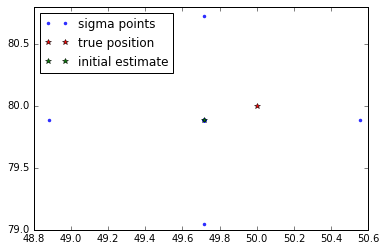#### Propagate Sigma Points¶

\begin{align} \underline{\chi}^i(k+1) &= {\bf{f}}(k, \underline{\chi}^i(k), \underline{u}(k), \underline{v}^i(k)) \end{align}
In :
def propagateSigmas(sigmas, noise):
new_sigmas = numpy.zeros((len(sigmas), 4))
for i in range(len(sigmas)):
new_sigmas[i,:] = f(sigmas[i,:] + noise[i,:])
return new_sigmas
new_sigmas = propagateSigmas(first_sigmas, first_noises)
plt.plot(new_sigmas[:,0], new_sigmas[:,1], 'b.', alpha=0.5, label='new sigmas')
plt.plot(first_sigmas[:,0], first_sigmas[:,1], 'b.', alpha=0.2, label='sigmas')
plt.plot(true_trajectory[0,0], true_trajectory[1,0], 'r*', alpha=0.5)
plt.plot(true_trajectory[0,1], true_trajectory[1,1], 'r*', label='true position')
plt.plot(x_start, x_start, 'g*', label='initial estimate')
plt.legend(loc='best')
plt.show()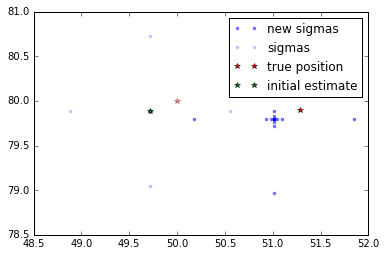#### Get Expected Measurement for each Sigma¶

\begin{align} \underline{\xi}^i &= {\bf{h}}(k+1,\underline{\chi}^i(k+1)) \end{align}
In :
def sigmaMeasurements(sigmas):
measurements = numpy.zeros(len(sigmas))
for i in range(len(sigmas)):
measurements[i] = h(sigmas[i,:])
return measurements
first_meas = sigmaMeasurements(new_sigmas)
plt.plot(first_meas, 'b.' , label='Expected Range Measurement')
plt.xlabel('Sigma Point Number')
plt.ylabel('Meters'); plt.title('Expected measurements')
plt.show()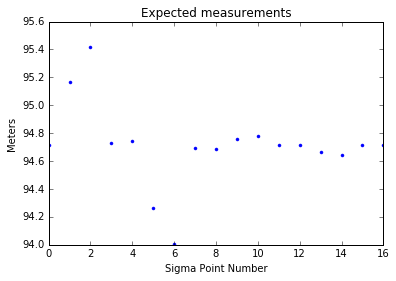#### Compute weighted mean and weighted mean measurement¶

\begin{align} \overline{x}(k+1) &= \sum_{i=0}^{2(n_x + n_v)}w_i^m \chi^i(k+1)\\ \overline{z}(k+1) &= \sum_{i=0}^{2(n_x + n_v)}w_i^m \xi^i(k+1) \end{align}
In :
def getMeans(sigmas, measurements, alpha=1, nx=4, nv=4):
kappa=3-nx
lam = alpha**2.*(nx+kappa) - nx
center_weight = lam/(nx+nv+lam)
other_weight = 1./(2*(nx+nv+lam))
x_mean = center_weight * sigmas[0,:]
z_mean = center_weight * measurements
for i in range(1,len(sigmas)):
x_mean += other_weight * sigmas[i,:]
z_mean += other_weight * measurements[i]
return x_mean, z_mean
xmean1, zmean1 = getMeans(new_sigmas, first_meas)
new_sigmas = propagateSigmas(first_sigmas, first_noises)
plt.plot(new_sigmas[:,0], new_sigmas[:,1], 'b.', alpha=0.5, label='new sigmas')
plt.plot(xmean1, xmean1, 'y*', label='x mean')
plt.plot(true_trajectory[0,1], true_trajectory[1,1], 'r*', label='true position')
plt.plot(x_start, x_start, 'g*', label='initial estimate')
plt.legend(loc='best')
plt.show()
plt.plot(first_meas, 'b.' , label='Expected Range Measurement')
plt.plot(len(first_meas)/2, zmean1, 'r*', label="Z_mean")
plt.xlabel('Sigma Point Number')
plt.ylabel('Meters'); plt.title('Expected measurements')
plt.show()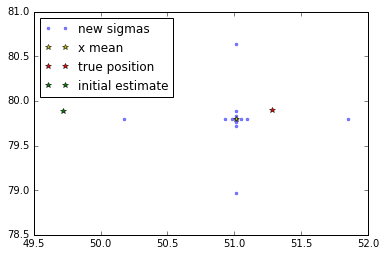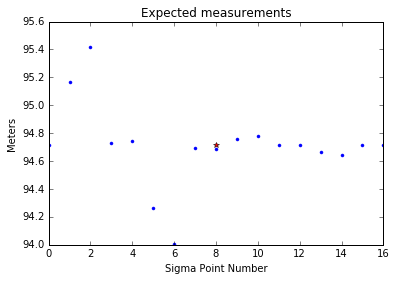#### Compute covariances¶

\begin{align} \overline{P}(k+1) &= \sum_{i=0}^{2(n_x+n_v)} w_i^c \left[\chi^i(k+1) - \overline{x}(k+1)\right]\left[\chi^i(k+1) - \overline{x}(k+1)\right]^T\\ \overline{P}_{xz}(k+1) &= \sum_{i=0}^{2(n_x+n_v)} w_i^c \left[\chi^i(k+1) - \overline{x}(k+1)\right]\left[\xi^i(k+1) - \overline{z}(k+1)\right]^T\\ \overline{P}_{zz}(k+1) &= \sum_{i=0}^{2(n_x+n_v)} w_i^c \left[\xi^i(k+1) - \overline{z}(k+1)\right]\left[\xi^i(k+1) - \overline{z}(k+1)\right]^T \end{align}
\begin{align} K &= \overline{P}_{xz}\overline{P}_{zz}^{-1} \end{align}
In :
def computeCovariances(xmean, zmean, sigmas, measurements, alpha=1, beta=2, nx=4, nv=4):
kappa=3-nx
lam = alpha**2.*(nx+kappa) - nx
center_weight = (lam/(nx+nv+lam)) + 1 - alpha**2. + beta
other_weight = 1./(2*(nx+nv+lam))

Pxx = center_weight * numpy.dot(numpy.array([sigmas[0,:] - xmean]).T,
numpy.array([sigmas[0,:] - xmean]))
Pxz = center_weight * numpy.dot(numpy.array([sigmas[0,:] - xmean]).T,
numpy.array([[measurements - zmean]]))
Pzz = center_weight * numpy.dot(numpy.array([[measurements - zmean]]).T,
numpy.array([[measurements - zmean]]))

for i in range(1, len(sigmas)):
Pxx += other_weight * numpy.dot(numpy.array([sigmas[i,:] - xmean]).T,
numpy.array([sigmas[i,:] - xmean]))
Pxz += other_weight * numpy.dot(numpy.array([sigmas[i,:] - xmean]).T,
numpy.array([[measurements[i] - zmean]]))
Pzz += other_weight * numpy.dot(numpy.array([[measurements[i] - zmean]]).T,
numpy.array([[measurements[i] - zmean]]))
Pzz += R
return Pxx, Pxz, Pzz
newPxx, newPxz, newPzz = computeCovariances(xmean1, zmean1, new_sigmas, first_meas)
print newPxx

[[  1.01109730e-01   1.75885882e-10   4.37023775e-03   6.49649874e-10]
[  1.75885882e-10   1.01109730e-01  -3.51771626e-10   4.37023789e-03]
[  4.37023775e-03  -3.51771626e-10   1.78363782e-02  -1.29929947e-09]
[  6.49649874e-10   4.37023789e-03  -1.29929947e-09   1.78363769e-02]]


#### Update Estimate¶

\begin{align} {\bf{\hat{x}}}(k+1) &= \overline{x}(k+1) + K\left[z(k+1) - \overline{z}(k+1)\right]\\ P(k+1) &= \overline{P}(k+1) - KRK^T \end{align}
In :
def updateEstimate(xmean, Pxx, Pxz, Pzz, zmean, time_index):
xnew = numpy.array([xmean]).T + numpy.dot(Pxz, pinv(Pzz))*(measurements[time_index] - zmean)
xnew = xnew.T
Pnew = Pxx - numpy.dot(Pxz, numpy.dot(Pzz, Pxz.T))
return xnew, Pnew
newx, newP = updateEstimate(xmean1, newPxx, newPxz, newPzz, zmean1, 1)
plt.plot(true_trajectory[0,0], true_trajectory[1,0], 'r*', alpha=0.5)
plt.plot(true_trajectory[0,1], true_trajectory[1,1], 'r*', label='true position')
plt.plot(x_start, x_start, 'g*', alpha=0.5, label='initial estimate')
plt.plot(newx, newx, 'g*', label='updated estimate')
plt.legend(loc='lower left')
plt.show()

---------------------------------------------------------------------------
NameError                                 Traceback (most recent call last)
<ipython-input-5-7252dda05bc0> in <module>()
4     Pnew = Pxx - numpy.dot(Pxz, numpy.dot(Pzz, Pxz.T))
5     return xnew, Pnew
----> 6 newx, newP = updateEstimate(xmean1, newPxx, newPxz, newPzz, zmean1, 1)
7 plt.plot(true_trajectory[0,0], true_trajectory[1,0], 'r*', alpha=0.5)
8 plt.plot(true_trajectory[0,1], true_trajectory[1,1], 'r*', label='true position')

NameError: name 'xmean1' is not defined

### Full Simulation¶

Run a UKF on the full trajectory

In :
def runUKF():
P = P0
x = x_start

P_array = numpy.zeros((len(t), 4, 4))
x_array = numpy.zeros((len(t), 4))
P_array = P
x_array[0,:] = x_start
for i in range(1, len(t)):
Sx, Sv = getCholesky(P, Q)
sigmas, noises = formSigmas(x, Sx, Sv)
propagated_sigmas = propagateSigmas(sigmas, noises)
expected_measurements = sigmaMeasurements(propagated_sigmas)
xmean, zmean = getMeans(propagated_sigmas, expected_measurements)
Pxx, Pxz, Pzz = computeCovariances(xmean, zmean, propagated_sigmas,
expected_measurements)
xnew, Pnew = updateEstimate(xmean, Pxx, Pxz, Pzz, zmean, i)

P_array[i] = Pnew
x_array[i,:] = xnew

P = Pnew
x = xnew
return x_array, P_array
ukfresults = runUKF()
ukf_traj, ukf_cov = ukfresults


### Plot Results¶

In :
def showUKFResults(trajectory, ukf_traj, ukf_cov):
plt.plot(trajectory[:,0], trajectory[:,1], label='true trajectory')
plt.plot(ukf_traj[:,0], ukf_traj[:,1], label='estimated trajectory')
plt.title('True and Estimated Projectile Trajectory')
plt.xlabel('meters'); plt.ylabel('meters')
plt.legend(loc='best')
plt.ylim([0,100])
plt.show()
plt.plot(t, trajectory[:,0], label='true')
plt.plot(t, ukf_traj[:,0], label='estimated')
plt.legend(loc='best')
plt.title('Projectile x-Position')
plt.xlabel('sec'); plt.ylabel('meters')
plt.show()
plt.plot(t, trajectory[:,1], label='true')
plt.plot(t, ukf_traj[:,1], label='estimated')
plt.legend(loc='best')
plt.title('Projectile y-Position')
plt.xlabel('sec'); plt.ylabel('meters')
plt.show()
plt.plot(t, trajectory[:,2], label='true')
plt.plot(t, ukf_traj[:,2], label='estimated')
plt.legend(loc='best')
plt.title('Projectile x-Velocity')
plt.xlabel('sec'); plt.ylabel('meters/sec')
plt.show()
plt.plot(t, trajectory[:,3], label='true')
plt.plot(t, ukf_traj[:,3], label='estimated')
plt.legend(loc='best')
plt.title('Projectile y-Velocity')
plt.xlabel('sec'); plt.ylabel('meters/sec')
plt.show()
showUKFResults(trajectory, ukf_traj, ukf_cov)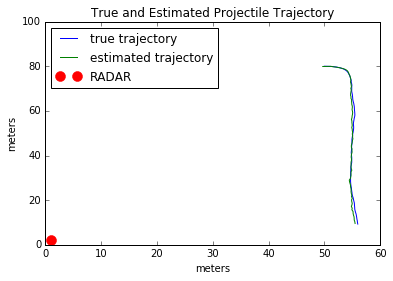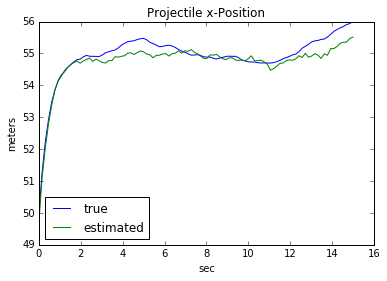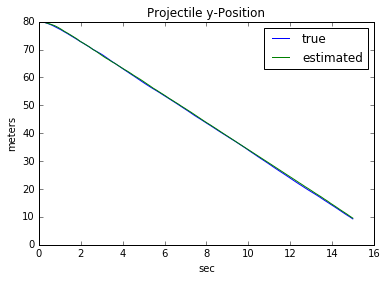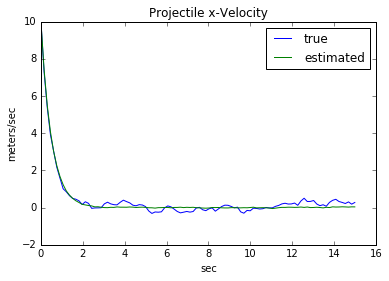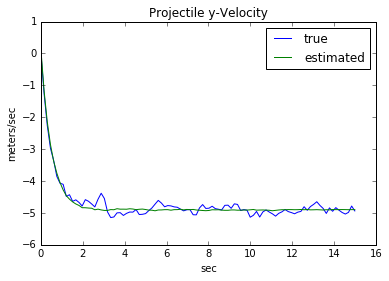### Finishing Thoughts¶

Pros: Significantly more efficient than the particle filter, can handle highly nonlinear dynamics and measurement models, can handle non-differentiable dynamics and measurement models, generally stable

Cons: Still slower than EKF's (but often more accurate), need to be wary of sigma point spacing

## The Extended Kalman Filter¶

### Intuition¶

For the sigma-point filter, we made assumptions about the underlying distributions driving the process noise and our uncertainty in the system. Let's add a few more assumptions.

Let us furthermore assume that the propagation and measurement equations are always differentiable (this is the case for us, it is not the case for any system involving bouncing or collisions), and let us assume that the uncertainty ellipse stays centered on the state estimate throughout the propagation step. In the sigma-point filter, we approximated the covariance numerically so we didn't require that the uncertainty stay centered (or, in practice, very-close-to-centered) on the state estimate.

If we make this assumption, than we can reduce the number of "particles" to just one. Rather than numerically estimate the covariance, we instead use the system dynamics to propagate it too. The price that you pay for this is an inability to capture the nonlinearities in the propagation and measurment functions (as you'll see, we essentially just Euler-integrate both of these, which doesn't work well on nonlinear functions). What we get in return, however, is speed.

### Overview of Algorithm¶

#### Propagate Estimate¶

Using the nonlinear state update equations, we propagate our current estimate for the state at time $k$ forward one timestep. This gives us our best guess (based on the dynamics, assuming no process noise) of what the state at the next timestep will be.

\begin{align} \hat{x}_{k+1}^- &= f(\hat{x}_k^+) \end{align}

#### Propagate Covariance¶

Unlike before, where we just numerically approximated the covariance, we instead use our dynamics model to also propagate the covariance matrix forward one timestep. In the equation below, $F$ is the Jacobian of the dynamics model with respect to the state, and $Q$ is the process noise error covariance.

\begin{align} P_{k+1}^- &= F(\hat{x}_k^+)P_{k}^+F(\hat{x}_k^+)^T + Q \end{align}

where

\begin{align} F(x_0) &= \left[\frac{\partial{}}{\partial{x}}f \right]_{x_0} \end{align}

It's worth pausing here for a moment to understand this equation. Why does this equation propagate the covariance? Let's suppose that the true value for the state at time $k$, $x_k$, is equal to our estimate for that state, $\hat{x}_k^+$, plus a small deviation, $\Delta x_k$.

\begin{align} x_k &= \hat{x}_k^+ + \Delta x_k \end{align}

We can use the nonlinear update equations and the above expression to find the true state at the next timestep, $k+1$:

\begin{align} x_{k+1} &= f(x_k)\\ &= f(\hat{x}_k^+ + \Delta x_k) \end{align}

We can use a Taylor Series to approximate this expression:

\begin{align} x_{k+1} &= f(\hat{x}_k^+ + \Delta x_k)\\ &\approx f(\hat{x}_k^+) + \left[\frac{\partial{}}{\partial{x}} f\right]_{\hat{x}_k^+}\Delta x_k\\ &\approx f(\hat{x}_k^+) +F(\hat{x}_k^+)\Delta x_k \end{align}

Subtract the constant term to the other side:

\begin{align} x_{k+1} - f(\hat{x}_k^+) &\approx F(\hat{x}_k^+)\Delta x_k\\ \Delta x_{k+1} &\approx F(\hat{x}_k^+)\Delta x_k \end{align}

The Jacobian, $F$, maps a small estimation error at time $k$ forward one timestep to $k+1$. But how does this relate to covariance propagation. We consider the small error $\Delta x$, to be a zero-mean Gaussian random number. The covariance can then be written in terms of these random numbers:

\begin{align} P^+_k &= E\left[\Delta x_{k} \Delta x_k^T\right]\\ P_{k+1}^- &= E\left[\Delta x_{k+1} \Delta x_{k+1}^T\right] \end{align}

Consider that bottom expression:

\begin{align} P^-_{k+1} &= E\left[\Delta x_{k+1} \Delta x_{k+1}^T\right]\\ &= E\left[F\Delta x_{k} \Delta x_{k}^TF^T\right]\\ &= FE\left[\Delta x_{k} \Delta x_{k}^T\right]F^T\\ &= FP^+_kF^T \end{align}

The last thing to do is add on the covariance contribution from the process noise, which always acts to increase our uncertainty in the state.

\begin{align} P_{k+1}^- &= F(\hat{x}_k^+)P_{k}^+F(\hat{x}_k^+)^T + Q \end{align}

#### Expected Measurement¶

By pumping our propagated state through the nonlinear measurement equations, we get the measurement that we expect to gather at the next timestep.

\begin{align} \hat{z}_{k+1} &= h(\hat{x}_{k+1}^-) \end{align}

#### Innovation Covariance¶

This expression should look familiar. This is exactly the same equation that we used to propagate the state covariance, but we have $H$ instead of $F$, and $R$ instead of $Q$. $H$ is the Jacobian of the measurement equations with respect to the state, and $R$ is the measurement error covariance matrix.

\begin{align} P_{zz} &= H(\hat{x}_{k+1}^-)P_{k+1}^-H(\hat{x}_{k+1}^-)^T + R \end{align}

where

\begin{align} H(x_0) &= \left[\frac{\partial{}}{\partial{x}}h \right]_{x_0} \end{align}

In our case:

\begin{align} z = h({\bf{x}}) = \sqrt{x^2 + y^2} + w \end{align}
\begin{align} H &= \begin{bmatrix} \frac{\partial{h_1}}{\partial{x}} & \frac{\partial{h_1}}{\partial{y}} & \frac{\partial{h_1}}{\partial{\dot{x}}} & \frac{\partial{h_1}}{\partial{\dot{y}}} \end{bmatrix}\\ &= \begin{bmatrix} \frac{x}{\sqrt{x^2 + y^2}} & \frac{y}{\sqrt{x^2 + y^2}} & 0 & 0\end{bmatrix} \end{align}

You should be able to guess where this comes from after the first derivation. But just to be explicit:

\begin{align} z_k &= h(x_k) + r_k\\ &= h(\hat{x}_k^- + \Delta x_k) + r_k\\ &\approx h(\hat{x}_k^-) + H\Delta x_k + r_k\\ z_k - \hat{z}_k &\approx H\Delta x_k + r_k\\ \Delta z_k &\approx H\Delta x_k + r_k \end{align}

And the covariance:

\begin{align} P_{zz} &= E\left[\Delta z_k \Delta z_k^T\right]\\ &= E\left[H\Delta x_k \Delta x_k^T H^T\right] + E\left[r_k r_k^T\right]\\ &= HE\left[\Delta x_k \Delta x_k^T\right]H^T + R\\ &= HP_k^-H^T + R \end{align}

#### State-Innovation Covariance¶

Using what we've done above, we can find the state innovation covariance:

\begin{align} P_{xz} &= P_k^-H(\hat{x}_{k+1})^T \end{align}

We know how to find these covariances now. Let's just plug in and solve to arrive at this equation.

\begin{align} P_{xz} &= E\left[\Delta x_k \Delta z_k^T\right]\\ &= E\left[\Delta x_k \left(\Delta x_k^T H^T + r^T\right)\right]\\ &= E\left[\Delta x_k \Delta x_k^T\right]H^T + E\left[\Delta x_k r^T\right]\\ &= E\left[\Delta x_k \Delta x_k^T\right]H^T + 0\\ &= P_k^- H^T \end{align}

#### Compute Kalman Gain¶

We haven't justified this as a good choice for the gain. When we get to the linear Kalman filter we will show that, for linear systems, this produces the optimal estimate. That's why we use it here.

\begin{align} K &= P_{xz}P_{zz}^{-1} \end{align}

#### Update State Estimate¶

The state correction is precisely the same as in the sigma point filter. The updated estimate is simply our propagated state, plus a correction. That correction is the difference between the expected and the true measurements, weighted by the Kalman gain. If the term in the parentheses is small (our expected measurement matches our true measurement), then the right-hand term will be near zero and we won't change our propagated state much.

Alternatively, if the term in the parantheses is large (big difference between true and expected measurement) and the Kalman gain is large (we trust our sensors), then we will correct the propagated term.

Finally, if the term in the parentheses is large (big measurement error), but $K$ is small (we don't trust our sensors), then we don't change the propagated state much, despite the large measurement error.

\begin{align} \hat{x}_{k+1}^+ &= \hat{x}_{k+1}^- + K(z_{k+1} - \hat{z}_{k+1}) \end{align}

#### Update Covariance Estimate¶

Finally, we update the covariance estimate:

\begin{align} P_{k+1}^+ &= P_{k+1}^- - KH(\hat{x}_{k+1}^-)P_{k+1}^- \end{align}

This expression can be derived using the same methods that we've been using. I'm going to use Tucker's notation.

Recall that $\Delta x$ is defined as the perturbation of the true state from the predicted state $\hat{x}_k^-$. So, if we want to talk about the perturbation of the corrected state $\hat{x}_k^+$ from the true state, then we need to define a new variable. Let's call that variable $\Delta x^+_k$.

\begin{align} x_k &= \hat{x}_k^+ + \Delta x_k^+ \end{align}

And recall that we have an expression for the corrected estimate:

\begin{align} \hat{x}_k^+ &= \hat{x}_k^- + K(z_k - \hat{z}_k)\\ &= \hat{x}_k^- + K\Delta z_k \end{align}

We can substitute this into the first expression:

\begin{align} \Delta x_k^+ &= x_k - \hat{x}_k^+\\ &= x_k - (\hat{x}_k^- + K\Delta z_k)\\ &= x_k - \hat{x}_k^- - K\Delta z_k\\ &= \Delta x_k - K \Delta z_k \end{align}

Now, as before, let us find the covariance of that variable:

\begin{align} P_k^+ &= E\left[\Delta x_k^+ \Delta x_k^{+^{T}}\right]\\ &= E\left[(\Delta x_k - K\Delta z_k)(\Delta x_k - K\Delta z_k)^T\right]\\ &= E\left[\Delta x_k \Delta x_k^T\right] - E\left[\Delta x_k \Delta z_k^T\right]K^T - KE\left[\Delta z_k \Delta x_k^T\right] + KE\left[\Delta z_k \Delta z_k^T\right] K^T \end{align}

Some of those terms look familiar! Let's substitute:

\begin{align} P_k^+ &= P_k^- - P_{xz}K^T - KP_{xz}^T + KP_{zz} K^T \end{align}

By the way, the expression above words for any gain $K$. We only care about the optimal one though, the Kalman Gain. So let's substitute that in only one location (just to help with simplification):

\begin{align} P_k^+ &= P_k^- - P_{xz}K^T - KP_{xz}^T + (P_{xz}P_{zz}^{-1})P_{zz} K^T\\ &= P_k^- - P_{xz}K^T - KP_{xz}^T + P_{xz} K^T\\ &= P_k^- - KP_{xz}^T \end{align}

And, finally, we have an expression for $P_{xz}$:

\begin{align} P_k^+ &= P_k^- - KP_{xz}^T \\ &= P_k^- - K(P_k^-H^T)^T\\ &= P_k^- - KHP_k^- \end{align}

There we have it. None of the math is hard, it's just algebraic manipulations.

### One Iteration¶

Walk through the first iteration of the EKF

#### Initial covariance and state estimate¶

\begin{align} \text{State Estimate} &= {\bf{\hat{x}}}(k)\\ \text{Coveriance Estimate} &= P(k)\\ \text{Process Noise} &\sim \mathcal{N}(0,Q(k))\\ \end{align}
In :
P0 = numpy.array([[.01, 0., 0., 0.],
[0., .01, 0., 0.],
[0., 0., .001, 0.],
[0., 0., 0., .001]])
x_start = x0 + numpy.random.multivariate_normal(numpy.zeros